# Common Core: High School - Geometry : Congruence

## Example Questions

### Example Question #1 : Represent And Compare Transformations In The Plane: Ccss.Math.Content.Hsg Co.A.2

Which one of the following best describes a reflection?

Slide

Extend

Shrink

Flip

None of the answers

Flip

Explanation:

To answer this question first recall the different geometric definitions.

Translation: To move an object from its original position a certain distance without changing the object in any other way.

Transformation: Refers to any of the four changes that can be done to an object geographically. Transformations include, reflections, translations, rotations, and resizing the object.

Reflection: To flip the orientation of an object over a specific line or function.

Rotation: To rotate an object either clockwise or counter clockwise around a center point.

Therefore, the English term that best represents reflection is "flip".

### Example Question #1 : Represent And Compare Transformations In The Plane: Ccss.Math.Content.Hsg Co.A.2

Which one of the following best describes a translation?

Extend

Flip

Turn

Slide

Shrink

Slide

Explanation:

To answer this question first recall the different geometric definitions.

Translation: To move an object from its original position a certain distance without changing the object in any other way.

Transformation: Refers to any of the four changes that can be done to an object geographically. Transformations include, reflections, translations, rotations, and resizing the object.

Reflection: To flip the orientation of an object over a specific line or function.

Rotation: To rotate an object either clockwise or counter clockwise around a center point.

Therefore, the English term that best represents translation is "slide".

### Example Question #21 : Congruence

Mrs. Johnson's classroom is organized in a grid pattern. In her classroom there arerows andcolumns of desks resulting in a total ofdesks. Which of the following depicts the resulting mathematical function of the situation, given Billy sits in desk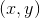and wants to movedesks to the south anddesk to the east.

Assume south represents a negative directional change in theaxis and east represents a positive directional change in theaxis.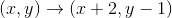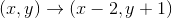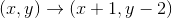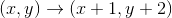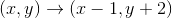Explanation:

To help solve this problem, look at the desks as a coordinate grid. Given that Billy currently sits in deskand assuming south represents a negative directional change in theaxis and east represents a positive directional change in theaxis, we can write the following transformation.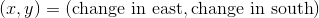Since Billy wants to move 2 desks south or in the "down" direction then the mathematical notation would be,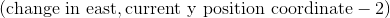Now since Billy also wants to move one desk to the east this results in the following,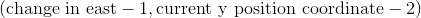From here, plug in the starting position which isand formulate the final transformation.### Example Question #22 : Congruence

Billy is flying a kite at the park. The kite starts touching the spool of string it is attached to. As Billy runs, the spool of string unravels and the kite fliesfeet in the air. Which geometric term describes this situation?

Rotation

Reflection

Dilation

Translation

None of the other answers

Translation

Explanation:

This question is applying mathematical and more specifically, geometric definitions to describe a real life situation. Geometric definitions usually correspond to objects in the 2 dimensional plane or 3 dimensional surface. Since the basket ball is the object in question, this problem is dealing with a the 3 dimensional space.

From here, recall what is given

"Billy is flying a kite at the park. The kite starts touching the spool of string it is attached to. As Billy runs, the spool of string unravels and the kite flies 20 feet in the air."

Recall that a translation is to move an object from its original position a certain distance without changing the object in any other way. Since the kit does not change size of shape but it does move a certain distance from where it originally started, this matches a translation.

Therefore, the correct answer is

"Translation"

### Example Question #1 : Rotations And Reflections Of Rectangles, Parallelograms, Trapezoids, And Regular Polygons: Ccss.Math.Content.Hsg Co.A.3

Determine whether the statement is true or false:

The following image can be divided multiple ways to result in a reflected image.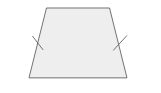False

True

False

Explanation:

Looking at the statement and the image given, it is seen that the object is a trapezoid.

A trapezoid has two bases of differing lengths and two side pieces of equal length in this particular case.For an object to be divided into images that can be reflected onto one another, the images must be identically mirrored.

To identify the possible solutions, draw the lines of symmetry.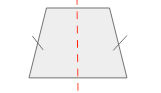Only one line of symmetry could be drawn to allow for two images of the trapezoid to be reflected over that line. The trapezoid cannot be divided in any other way that will result in a reflected image.

Therefore, the original statement is false.

### Example Question #1 : Rotations And Reflections Of Rectangles, Parallelograms, Trapezoids, And Regular Polygons: Ccss.Math.Content.Hsg Co.A.3

Given a hexagon, how many lines of symmetry exist?

Twelve

Three

Two

Four

Six

Six

Explanation:

Recall that a hexagon is a six sided figure. Now recall that a line of symmetry creates two mirrored, identical figures which is also known as a reflection.

Knowing these two pieces of information draw the image of the hexagon and the lines of symmetry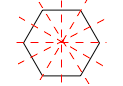Counting up these lines results 6 lines of symmetry.

Therefore, the answer is 6.

### Example Question #2 : Rotations And Reflections Of Rectangles, Parallelograms, Trapezoids, And Regular Polygons: Ccss.Math.Content.Hsg Co.A.3

A cylinder is composed of two circular bases. For one base to be carried onto the other, what geometric transformation must occur?

Translation

Extension

Rotation

Rotation and Translation

All of the answers are correct.

Translation

Explanation:

Since a cylinder is composed of two, identical circular bases that are separated by the height of the cylinder, the transformation that must occur to have one carried onto the other is a translation. Recall that a translation is the sliding of an object without changing its size or shape. Therefore, for the one base to be carried onto the other, translation is the geometric transformation that must occur.

### Example Question #3 : Rotations And Reflections Of Rectangles, Parallelograms, Trapezoids, And Regular Polygons: Ccss.Math.Content.Hsg Co.A.3

If the rectangle is reflected across the x-axis, what is the resulting image?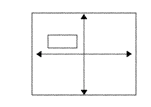None of the other answers.Explanation:

In order to create the resulting image of a reflection first recall what a reflection is.

Reflection: To flip the orientation of an object over a specific line or function.

In this specific situation the line of reflection is the x-axis.

The original image is,When the image is reflected across the x-axis result in,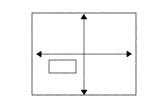This is the correct answer.

### Example Question #3 : Rotations And Reflections Of Rectangles, Parallelograms, Trapezoids, And Regular Polygons: Ccss.Math.Content.Hsg Co.A.3

Given a trapezoidal based prism how can one base be carried onto the other?

Reflection

Translation

Extension

None of the answers.

Rotation

Translation

Explanation:

Since a trapezoidal based prism is composed of two, identical trapezoidal bases that are separated by the height of the prism, the transformation that must occur to have one base carried onto the other is a translation. Recall that a translation is the sliding of an object without changing its size or shape. Therefore, for the one base to be carried onto the other, translation is the geometric transformation that must occur.

### Example Question #4 : Rotations And Reflections Of Rectangles, Parallelograms, Trapezoids, And Regular Polygons: Ccss.Math.Content.Hsg Co.A.3

Determine whether the statement is true or false:

Reflections and rotations never result in the same image.

True

False

False

Explanation:

To determine whether the statement is true or false, identify any example that would make the statement false.

"Reflections and rotations never result in the same image."

Imagine a square that exists in quadrant two. When this image is reflected across the y-axis it is still a square that is now in quadrant one. When the square from quadrant two is rotated around the origin, it results in the same image in quadrant one. Therefore, it is possible for a reflection and rotation to result in the same image.

Thus, the statement "Reflections and rotations never result in the same image." is false.

### All Common Core: High School - Geometry Resources